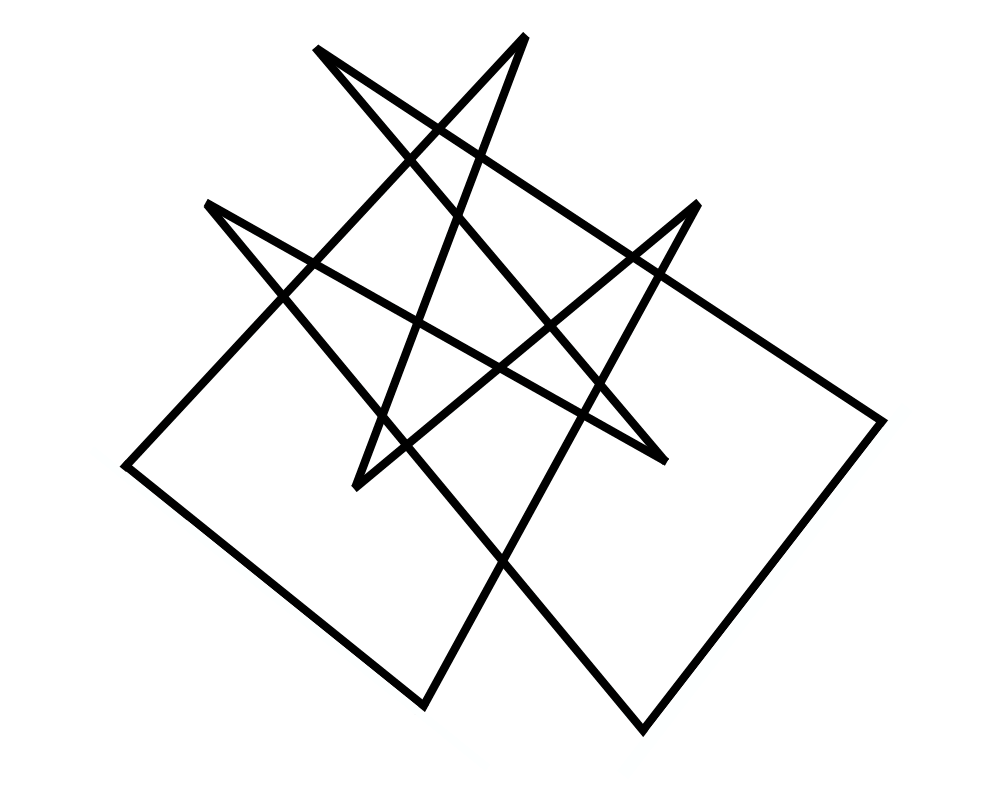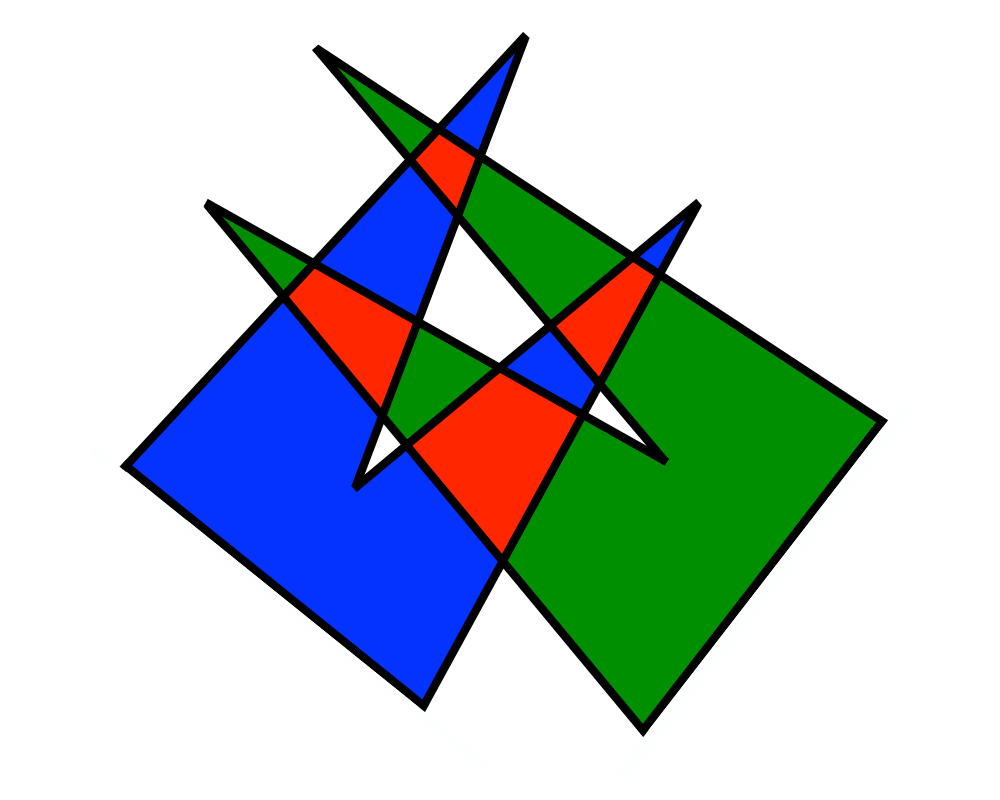# Intersection DetectionInputOutput

Input Description: A set $$S$$ of lines and line segments $$l_1,...,l_n$$, or a pair of polygons or polyhedra $$P_1$$ and $$P_2$$.
Problem: Which pairs of line segments intersect each other? What is the intersection of $$P_1$$ and $$P_2$$?

Excerpt from The Algorithm Design Manual: Intersection detection is a fundamental geometric primitive that arises in many applications. Picture a virtual-reality simulation of an architectural model for a building. The illusion of reality vanishes the instant the virtual person walks through a virtual wall. To enforce such physical constraints, any such intersection between polyhedral models must be immediately detected and the operator notified or constrained.

Another application of intersection detection is design rule checking for VLSI layouts. A minor design mistake resulting in two crossing metal strips can short out the chip, but such errors can be detected before fabrication using programs to find all intersections between line segments.

Go To Main Page